# Navier-Stokes equations

(diff) ← Older revision | Latest revision (diff) | Newer revision → (diff)

## Continuity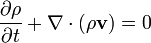$\frac{\partial \rho}{\partial t} + \nabla \cdot (\rho \mathbf{v}) = 0$

or, using the substantive derivative: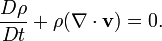$\frac{D\rho}{Dt} + \rho (\nabla \cdot \mathbf{v}) = 0.$

For an incompressible fluid,$\rho$ is constant, hence the velocity field must be divergence-free: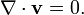$\nabla \cdot \mathbf{v} =0.$

## Momentum

(Also known as the Navier-Stokes equation.)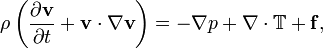$\rho \left(\frac{\partial \mathbf{v}}{\partial t} + \mathbf{v} \cdot \nabla \mathbf{v} \right) = -\nabla p + \nabla \cdot\mathbb{T} + \mathbf{f},$

or, using the substantive derivative: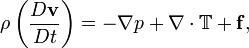$\rho \left(\frac{D \mathbf{v}}{D t} \right) = -\nabla p + \nabla \cdot\mathbb{T} + \mathbf{f},$

where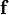$\mathbf{f}$ is a volumetric force (e.g.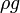$\rho g$ for gravity), and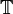$\mathbb{T}$ is the stress tensor.

Another form of the equation, more similar in form to the continuity equation, stresses the fact that the momentum density is conserved. For each of the three Cartesian coordinates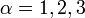$\alpha=1,2,3$: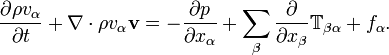$\frac{\partial \rho v_\alpha}{\partial t} + \nabla \cdot {\rho v_\alpha \mathbf{v}} = -\frac{\partial p}{\partial x_\alpha} + \sum_\beta \frac{\partial }{\partial x_\beta} \mathbb{T}_{\beta\alpha} + f_\alpha.$

In vector form: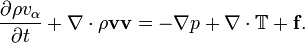$\frac{\partial \rho v_\alpha}{\partial t} + \nabla \cdot {\rho \mathbf{v} \mathbf{v}} = -\nabla p + \nabla\cdot\mathbb{T} + \mathbf{f}.$

The term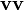$\mathbf{v} \mathbf{v}$ is a dyad (direct tensor product).

## Stress

The vector quantity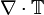$\nabla \cdot\mathbb{T}$ is the shear stress. For a Newtonian incompressible fluid,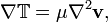$\nabla \mathbb{T} = \mu \nabla^2 \mathbf{v},$

with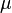$\mu$ being the (dynamic) viscosity.

For an inviscid fluid, the momentum equation becomes Euler's equation for ideal fluids: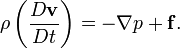$\rho \left(\frac{D \mathbf{v}}{D t} \right) = -\nabla p + \mathbf{f} .$